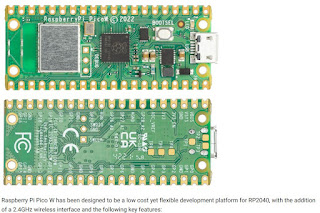## ShopShowing posts with label MATLAB. Show all posts
Showing posts with label MATLAB. Show all posts

## Saturday, September 25, 2021

### MATLAB - Family of Curves (Vectorization)

Linear Signals and System, matrix operation fundamentals. This example illustrates a techniques called vectorization. Algorithm vectorization uses matrix and vector operations to avoid manual repetition and loop structures. With simultaneous creation of curves one can maximize the use of matrix applications.
The result here is a 201 x 11 matrix with identical columns.

%% Matrix Operations - Family of Curves
r = [1 0 0]
A = [2 3;4 5;0 6]
c = r' B = [c A]
B(1,2)
B(1:2,2:3)
B(2,:)
A = [1 -2 3;-sqrt(3) 1 -sqrt(5);3 -sqrt(7) 1];
y = [1;pi;exp(1)];
x = inv(A)*y x1 = det([y,A(:,2:3)])/det(A) alpha = (0:10);
t = (0:0.001:0.2)';
T = t*ones(1,11);
H = exp(-T*diag(alpha)).*sin(2*pi*10*T+pi/6);
plot(t,H); xlabel('t'); ylabel('h(t)');
[R,P,K] = residue(B,A)
[R,P,K] = residue([1 0 0 0 0 pi],[1 -sqrt(8) 0 sqrt(32) -4]);
R.', P.', K title('Family of Curves - Vectorization')

Try this code out to graph this figure! Modify freely and comment on this post if it helps. Thanks for reading.

## Sunday, May 26, 2019

### Matlab on iPhone & iPad Devices

Written by Larsha Johnson
5/26/2019

Do you want to use your iPhone or iPad to collect data from built in sensors such as acceleration, angular velocity, orientation, magnetic field, and GPS?

In this blog I will explain how to use MATLAB to collect data and log it.

Once all downloads have been made, open the mobile app and press the icon at the bottom right labeled 'More'. There you will see the Sensors option. Press it. This opens the menu for five (5) different built-in sensors. To begin, turn on one or more sensors. After the selection has been made, you can stream to MATLAB or log the data.

Next, press the start button to collect data from your iOS device. *Before getting started you can change the frequency. If you chose to log the data, access the new file by pressing the icon to the right of the start button. See screenshots below.